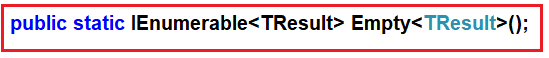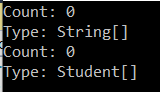# Linq Empty Method in C#

## Linq Empty Method in C# with Examples

In this article, I am going to discuss the LINQ Empty Method in C# with some examples. Please read our previous article before proceeding to this article where we discussed the LINQ Repeat Method with an example.

##### Linq Empty Method in C#:

The Empty Method belongs to the Generation Operator category. It is a static method included in the static Enumerable class. The LINQ Empty Method is used to return an empty collection (i.e. IEnumerable<T>) of a specified type. The following is the signature of this method.Here TResult specifies the type parameter of the returned generic IEnumerable<TResult>. This method returns an empty IEnumerable<TResult> whose type argument is TResult.

Note: The main advantage of using the Empty method is “Even if you use an empty array or empty collection, then those are objects. As objects, they are going to be stored in the memory. Once they stored in memory, then it is the responsibility of Garbage Collector to take care of them”.

##### Example:

In the following example, we have created two empty collections using the LINQ Empty Method. The first Empty method returns an empty collection of string while the second Empty method returns an empty collection of students.

```using System.Linq;
using System;
namespace LinqDemo
{
class Program
{
static void Main(string[] args)
{
var emptyCollection1 = Enumerable.Empty<string>();
var emptyCollection2 = Enumerable.Empty<Student>();

Console.WriteLine("Count: {0} ", emptyCollection1.Count());
Console.WriteLine("Type: {0} ", emptyCollection1.GetType().Name);

Console.WriteLine("Count: {0} ", emptyCollection2.Count());
Console.WriteLine("Type: {0} ", emptyCollection2.GetType().Name);

}
}

public class Student
{
public int ID { get; set; }
public string Name { get; set; }
}
}
```

Output:##### Why do we need the LINQ Empty Method?

Let us understand the need for Empty Method with an example.

Consider one scenario, where our application calls a method which returns an IEnumerable<int>. There might be a situation where the method returns null. In the following example, the GetData() method is returning null. So, when we run the following program, it will throw NULL reference exception.

```using System.Collections.Generic;
using System;
namespace LinqDemo
{
class Program
{
static void Main(string[] args)
{
IEnumerable<int> integerSequence = GetData();
foreach (var num in integerSequence)
{
Console.WriteLine(num);
}
}

private static IEnumerable<int> GetData()
{
return null;
}
}
}
```

The above problem can be fixed in two ways.

##### Solution1:

We need to check for NULL before looping through the items in the collection as shown in the below example.

```using System.Collections.Generic;
using System;
namespace LinqDemo
{
class Program
{
static void Main(string[] args)
{
IEnumerable<int> integerSequence = GetData();
if(integerSequence != null)
{
foreach (var num in integerSequence)
{
Console.WriteLine(num);
}
}
}

private static IEnumerable<int> GetData()
{
return null;
}
}
}```
##### Solution2:

We can solve the problem by using the LINQ Empty Method as shown in the below example. Here, we are using the NULL-COALESCING operator which checks if the GetData() method returns NULL, then initialized the integerSequence variable with an empty IEnumerable<int>.

```using System.Linq;
using System.Collections.Generic;
using System;
namespace LinqDemo
{
class Program
{
static void Main(string[] args)
{
IEnumerable<int> integerSequence = GetData() ?? Enumerable.Empty<int>(); ;

foreach (var num in integerSequence)
{
Console.WriteLine(num);
}